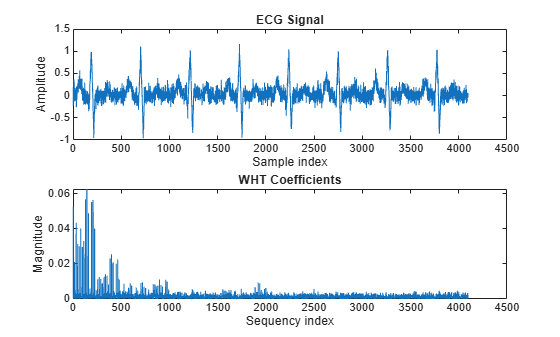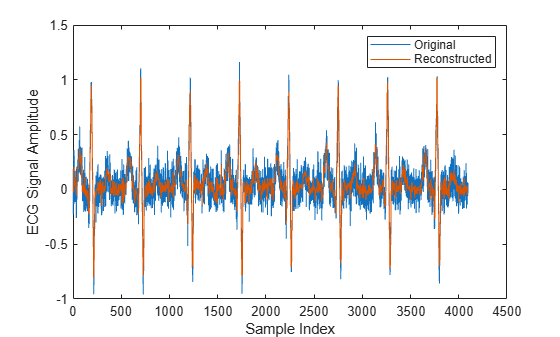# ifwht

## Syntax

``y = ifwht(x)``
``y = ifwht(x,n)``
``y = ifwht(x,n,ordering)``

## Description

example

````y = ifwht(x)` returns the coefficients of the inverse discrete fast Walsh-Hadamard transform of the input signal `x`.```
````y = ifwht(x,n)` returns the `n`-point inverse discrete Walsh-Hadamard transform, where `n` must be a power of 2.```
````y = ifwht(x,n,ordering)` specifies the ordering to use for the returned inverse Walsh-Hadamard transform coefficients.```

## Examples

collapse all

Use an electrocardiogram (ECG) signal to illustrate working with the Walsh-Hadamard transform. ECG signals typically are very large and need to be stored for analysis and retrieval at a future time. Walsh-Hadamard transforms are particularly well-suited to this application because they provide compression and thus require less storage space. They also provide rapid signal reconstruction.

Start with an ECG signal. Replicate it to create a longer signal and insert some additional random noise.

```xe = ecg(512); xr = repmat(xe,1,8); x = xr + 0.1.*randn(1,length(xr));```

Transform the signal using the fast Walsh-Hadamard transform. Plot the original signal and the transformed signal.

```y = fwht(x); subplot(2,1,1) plot(x) xlabel('Sample index') ylabel('Amplitude') title('ECG Signal') subplot(2,1,2) plot(abs(y)) xlabel('Sequency index') ylabel('Magnitude') title('WHT Coefficients')```The plot shows that most of the signal energy is in the lower sequency values, below approximately 1100. Store only the first 1024 coefficients (out of 4096). Try to reconstruct the signal accurately from only these stored coefficients.

```y(1025:length(x)) = 0; xHat = ifwht(y); figure plot(x) hold on plot(xHat) xlabel('Sample Index') ylabel('ECG Signal Amplitude') legend('Original','Reconstructed')```The reproduced signal is very close to the original but has been compressed to a quarter of the size. Storing more coefficients is a tradeoff between increased resolution and increased noise, while storing fewer coefficients can cause loss of peaks.

## Input Arguments

collapse all

Input signal, specified as a vector or matrix. If `x` is a matrix, the inverse fast Walsh-Hadamard transform is calculated on each column of `x`. The function operates only on signals with length equal to a power of 2. If the length of `x` is less than a power of 2, the function pads `x` with zeros to the next greater power of two before processing.

Data Types: `single` | `double`

Number of points in inverse discrete Walsh-Hadamard transform, specified as a positive even integer scalar. `n` must be a power of 2.

Data Types: `single` | `double` | `int8` | `int16` | `int32` | `int64` | `uint8` | `uint16` | `uint32` | `uint64`

Order of Walsh-Hadamard transform coefficients, specified as one of these:

OrderingDescription
`"sequency"`Coefficients in order of ascending sequency value, where each row has an additional zero crossing. This is the default ordering.
`"hadamard"`Coefficients in normal Hadamard order.
`"dyadic"`Coefficients in Gray code order, where a single bit change occurs from one coefficient to the next.

To specify the ordering, you must enter a value for the length `n` or, to use the default behavior, specify an empty vector (`[]`) for `n`. For more information on the Walsh functions and ordering, see Walsh-Hadamard Transform.

## Algorithms

The inverse fast Walsh-Hadamard transform algorithm is similar to the Cooley-Tukey algorithm used for the inverse FFT. Both use a butterfly structure to determine the transform coefficients. For more details, see the references.

 Beauchamp, Kenneth G. Applications of Walsh and Related Functions: With an Introduction to Sequency Theory. London: Academic Press, 1984.

 Beer, Tom. “Walsh Transforms.” American Journal of Physics. Vol. 49, 1981, pp. 466–472.